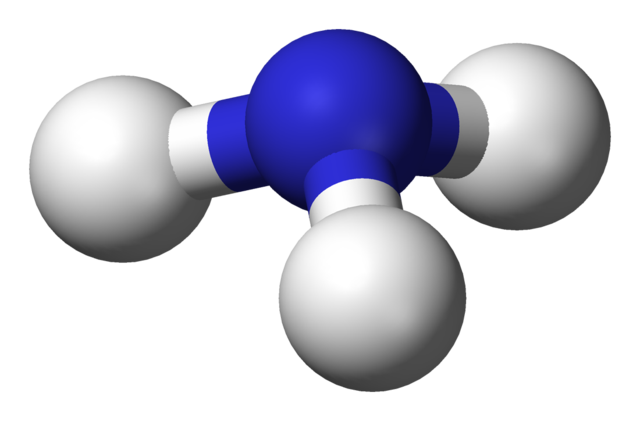GCSE Chemistry only - not for combined (double) science.

1. Which statement is always true about a reaction in dynamic equilibrium?

• A.   The concentration of reactants is equal to the concentration of products
• B.   The concentration of products is greater than the concentration of reactants
• C.   The forward and reverse reactions are occurring at the same rate
• D.   The forward and reverse reactions have both stopped

Q2-5: Ammonia is produced industrially in the Haber Process. The reaction is exothermic.

N2(g)   +   3H2(g)2NH3(g)

The process operates at a temperature of 4500C and a pressure of 200 atm.2. The reason that a higher temperature than 450°C is NOT used in this process is because ...
• A.   the equilibrium would move to the left reducing the amount of ammonia
• B.   the equilibrium would move to the right increasing the amount of ammonia
• C.   the rate of reaction would be slower at a higher temperature.
• D.   the rate of reaction would be faster at a higher temperature.
3. The reason that a lower temperature than 450°C is NOT used in this process is because ...
• A.   the equilibrium would move to the left reducing the amount of ammonia
• B.   the equilibrium would move to the right increasing the amount of ammonia
• C.   the rate of reaction would be too slow at a lower temperature.
• D.   the rate of reaction would be too fast at a lower temperature.

4. What would happen to the rate at which equilibrium is reached if a pressure of 100 atm was used instead of 200 atm and if a catalyst was added?

 Pressure 100 atm Catalyst added A increase decreases B increases increases C decreases decreases D decreases increases
5. Why is a pressure of 1000 atm NOT used in the Haber Process?
• A.   The equilibrium would move to the right increasing the amount of ammonia
• B.   The equilibrium would move to the left decreasing the amount of ammonia
• C.   High pressures are expensive to maintain
• D.   The rate of reaction would increase
6. A catalyst does not affect the position of equilibrium in a reversible reaction because catalysts ...
• A.   increase the rate of both reactions equally
• B.   only increase the rate of the forward reaction
• C.   only increase the rate of the reverse reaction
• D.   do not affect the rate of equilibrium reactions

7. Temperature can affect both the rate of reaction and the position of equilibrium.

The following reaction between methane and steam is endothermic.

CH4(g) + 2H2O(g)CO2(g) + 4H2(g)

What happens when the temperature of this reaction is increased?

 Rate of Reaction Position of equilibrium A increases Moves to the right B increases Moves to the left C decreases Moves to the right D decreases Moves to the left

8. Which essential elements for the promotion of plant growth are contained in the fertilizer ammonium phosphate, (NH4)3PO4?

• A.   nitrogen and hydrogen
• B.   nitrogen and phosphorus
• C.   phosphorus and oxygen
• D.   nitrogen, phosphorus and oxygen

Q9-10: The fertilizer ammonium nitrate can be produced by reacting ammonia with nitric acid.

9. What type of chemical is ammonium nitrate?
• A.   an acid
• B.   a salt
• C.   an alkali
• D.   a fuel
10. What type of reaction is taking place?
• A.   displacement
• B.   oxidation
• C.   neutralization
• D.   combustion